## Length

Length is a physical measurement of distance that is fundamentally measured in the SI unit of a meter.

### Learning Objectives

Distinguish SI and customary units of length

### Key Takeaways

#### Key Points

• The SI unit for length is the meter.
• One meter is defined as the distance that light travels in a vacuum in $\displaystyle \frac{1}{299,792,458}$ of a second.
• Derivatives of measurement units related to the meter are devised around the convenience of the number 10.

#### Key Terms

• Length: How far apart objects are physically.

Length can be defined as a measurement of the physical quantity of distance. Many qualitative observations fundamental to physics are commonly described using the measurement of length. The distance between objects, the rate at which objects are traveling, and how much force an object exerts are all dependent on length as a variable. In order to describe length in a standardized and quantitative manner, an accepted unit of measurement must be utilized.

Many different units of length are used around the world. In the United States, the U.S. customary units operationally describe length in terms of the basic unit of an inch. Varying lengths are thus described in relation to the inch, such as a foot equaling 12 inches, a yard equaling three feet, and a mile equaling 1,760 yards.

Though regional use of different measurement units is not generally problematic, it can raise issues of compatibility and understanding when working abroad or collaboratively with international partners. As such, a standard unit of measurement that is internationally accepted is needed. The basic unit of length as identified by the International System of Units (SI) is the meter. The meter is expressed more specifically in terms of speed of light.

One meter is defined as the distance that light travels in a vacuum in $\displaystyle \frac{1}{299,792,458}$ of a second. All lengths are measured in terms related to the meter, where its multiples are devised around the convenience of the number 10. For example, a centimeter is equal to $\displaystyle \frac{1}{100}$ of a meter (or $10^{-2}$ meters), and a kilometer is equal to 1,000 meters (or $10^{3}$ meters).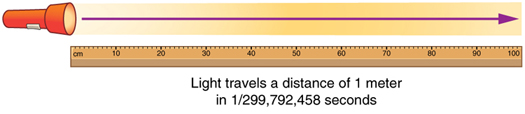Meter Defined by Speed of Light: The meter is defined to be the distance that light travels in 1/299,792,458 of a second in a vacuum. Distance traveled is speed multiplied by time.

Metric System – Length: A brief introduction to the metric system and unit conversions.

## Mass

Mass is the quantity of matter that an object contains, as measured by its resistance to acceleration.

### Learning Objectives

Explain the difference between mass and weight

### Key Takeaways

#### Key Points

• The kilogram is the only SI unit directly defined by the artifact itself.
• Mass is a property that does not depend on gravitational fields, unlike weight.
• One kilogram is defined as the mass of the International Prototype Kilogram (IPK), a platinum-iridium alloy cylinder.
• One kilogram is almost exactly equal to the mass of one liter of water.

#### Key Terms

• acceleration: the rate at which the velocity of a body changes with time
• inertia: the tendency of an object to resist any change in its motion

### Mass

Mass, specifically inertial mass, is a quantitative measure of an object’s resistance to acceleration. It is an intrinsic property of an object and does not change because of the environment. The SI unit of mass is the kilogram (kg).

The kilogram is defined as being equal to the mass of the International Prototype Kilogram (IPK), which is almost exactly equal to the mass of one liter of water. It is also the only SI unit that is directly defined by an artifact, rather than a fundamental physical property that can be reproduced in different laboratories. Four of the seven base units in the SI system are defined relative to the kilogram, so the stability of this measurement is crucial for accurate and consistent measurements.

In 2005, the International Committee for Weights and Measures (CIPM) recommended that the kilogram be redefined in terms of a fundamental constant of nature, due to evidence that the International Prototype Kilogram will vary in mass over time. At its 2011 meeting, the General Conference on Weights and Measures (CGPM) agreed that the kilogram should be redefined in terms of the Planck constant. The conference deferred a final decision until its next meeting in 2014.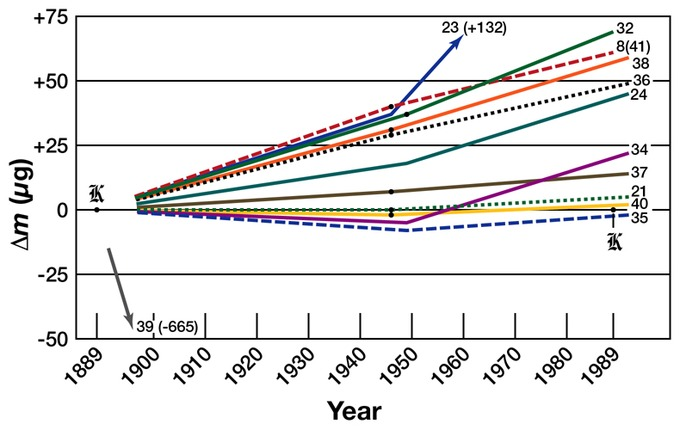Prototype Mass Drifts: A graph of the relative change in mass of selected kilogram prototypes.

### Mass and Weight

In everyday usage, the mass of an object in kilograms is often referred to as its weight. This value, though given in kilograms, is actually the non-SI unit of measure known as the kilogram- force. In scientific terms, ‘weight’ refers to the gravitational force acting on a given body. This measurement changes depending on the gravitational pull of the opposing body. For example, a person’s weight on the Earth is different than a person’s weight on the moon because of the differences in the gravitational pull of each body. In contrast, the mass of an object is an intrinsic property and remains the same regardless of gravitational fields. Accordingly, astronauts in microgravity must exert 10 times more force to accelerate a 10-kg object at the same rate as a 1-kg object, even though the differences in weight are imperceptible.

Metric System – Mass: A brief introduction to the metric system and unit conversions.

## Time

Time is the fundamental physical quantity of duration and is measured by the SI Unit known as the second.

### Learning Objectives

Relate time with other physical quantities

### Key Takeaways

#### Key Points

• Time is a physical quantity of duration.
• The SI Unit for time is the second.
• The second is operationally defined in terms of radiation emitted by cesium atoms.

#### Key Terms

• Radiation: the emission of energy as electromagnetic waves or as moving or oscillating subatomic particles.

Time is one of the seven fundamental physical quantities in the International System (SI) of Units. Time is used to define other quantities, such as velocity or acceleration, and as such, it is important that it be standardized and quantified precisely. An operational definition of time is highly useful in the conduct of both advanced experiments and everyday affairs of life.

Historically, temporal measurement was a prime motivation in navigation and astronomy. Periodic events and motion have long served as standards for units of time. For example, the movement of the sun across the sky, the phases of the moon, the swing of a pendulum, and the beat of a heart have all been used as a standard for time keeping. These events and standards, however, are highly dynamic in nature and cannot reliably be utilized for accurate quantitative measures. Between 1000 and 1960 the second was defined as $\displaystyle \frac{1}{86,400}$ of a mean solar day. This definition changed between 1960 and 1967 and was defined in terms of the period of the Earth’s orbit around the Sun in 1900. Today, the SI Unit of the second is defined in terms of radiation emitted by cesium atoms.

The second is now operationally defined as “the duration of 9,192,631,770 periods of the radiation corresponding to the transition between the two hyperfine levels of the ground state of the cesium 133 atom.” It follows that the hyperfine splitting in the ground state of the cesium 133 atom is exactly 9,192,631,770 hertz. In other words, cesium atoms can be made to vibrate in a very steady way, and these vibrations can be readily observed and counted. The second is the time required for 9,192,631,770 of these vibrations to occur.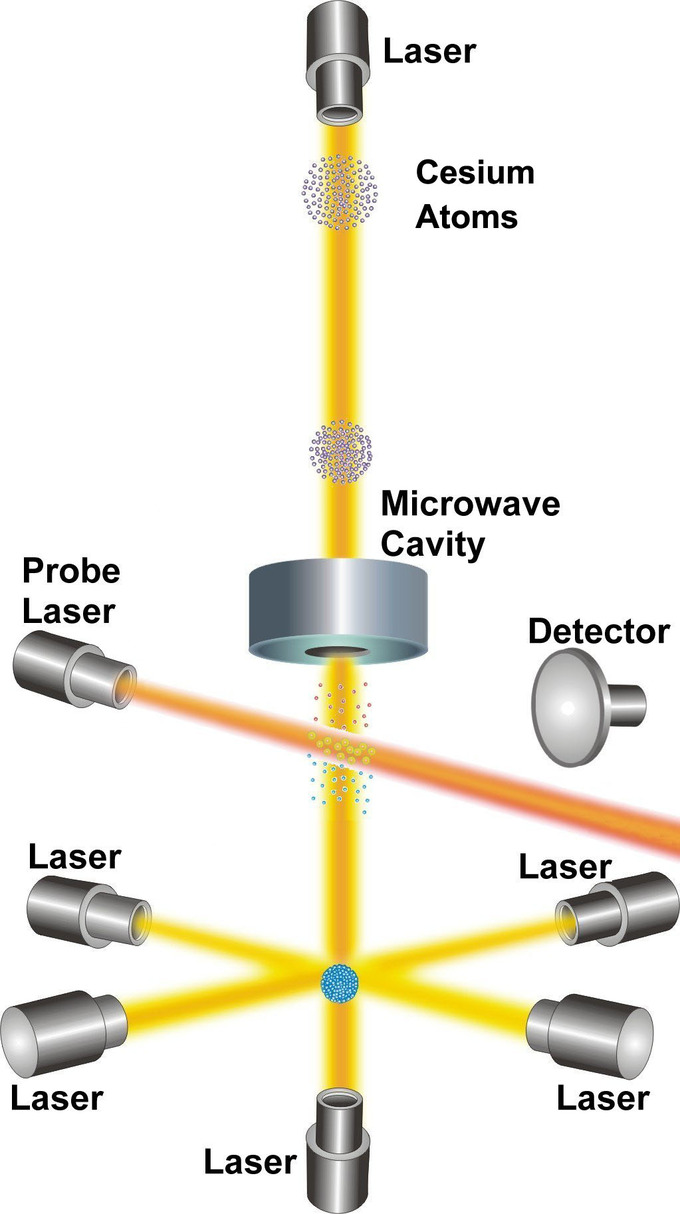NIST-F1 Cesium Clock: NIST-F1 is referred to as a fountain clock because it uses a fountain-like movement of atoms to obtain its improved reckoning of time.

Metric System – Time: A brief introduction to the metric system and unit conversions.

## Prefixes and Other Systems of Units

SI prefixes precede a basic unit of measure to indicate a multiple or fraction of the unit.

### Learning Objectives

Apply prefixes to units and distinguish between SI and customary units

### Key Takeaways

#### Key Points

• The twenty standardized prefixes for use in the International System of Units are derived from multiples of 10.
• The kilogram is the only SI unit with a prefix as part of its name and symbol; as such, SI unit prefixes are prepended to the unit gram.
• The United States customary units define measurements based on the English, or Imperial, unit standards.

#### Key Terms

• prefix: That which is prefixed; especially one or more letters or syllables added to the beginning of a word to modify its meaning; as, pre- in prefix, con- in conjure.

### Prefixes

A metric prefix, or SI prefix, is a unit prefix that precedes a basic unit of measure to indicate a multiple or fraction of the unit. Each prefix has a unique symbol that is prepended to the unit symbol. The prefix kilo-, for example, may be added to gram to indicate multiplication by one thousand; one kilogram is equal to one thousand grams (1 kg = 1000 g). The prefix centi-, likewise, may be added to meter to indicate division by one hundred; one centimeter is equal to one hundredth of a meter (1 cm = 0.01 m). Prefixes in varying multiples of 10 are a feature of all forms of the metric system, with many dating back to the system’s introduction in the 1790s. Today, the prefixes are standardized for use in the International System of Units (SI) by the International Bureau of Weights and Measures.There are twenty prefixes officially specified by SI.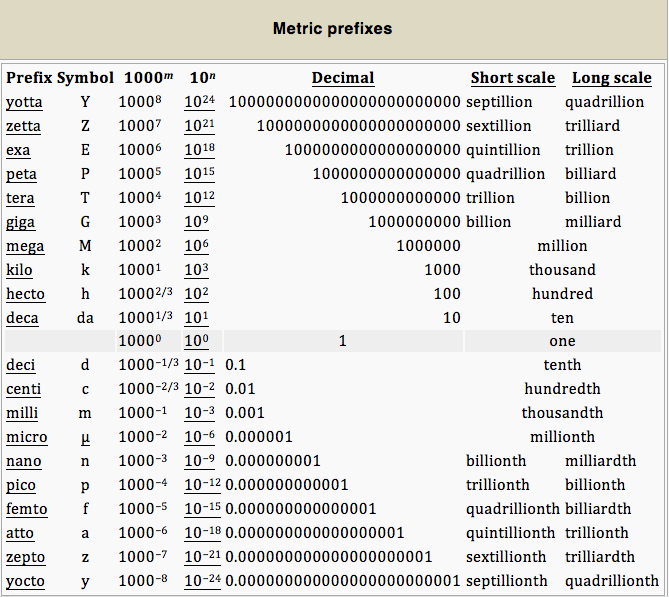SI Unit Prefixes: The twenty prefixes officially specified by the International System of Units

It is important to note that the kilogram is the only SI unit with a prefix as part of its name and symbol. Because multiple prefixes may not be used, in the case of the kilogram the prefix names are used with the unit name “gram” and the prefix symbols are used with the unit symbol “g. ” With this exception, any SI prefix may be used with any SI unit, including the degree Celsius and its symbol °C.

### Other Systems of Units

The SI Unit system, or the metric system, is used by the majority of countries in the world, and is the standard system agreed upon by scientists and mathematicians. Colloquially, however, other systems of units are used in many countries. The United States, for example, teaches and uses the United States customary units. This system of units was developed from the English, or Imperial, unit standards of the United Kingdom.The United States customary units define measurements using different standards than those used in SI Units. The system for measuring length using the United States customary system is based on the inch, foot, yard, and mile. Likewise, units of area are measured in terms of square feet, and units of capacity and volume are measured in terms of cubic inches, cubic feet, or cubic yards. Units of mass are commonly defined in terms of ounces and pounds, rather than the SI unit of kilograms.Other commonly used units from the United States customary system include the fluid volume units of the teaspoon, tablespoon, fluid ounce, US cup, pint, quart, and gallon, as well as the degrees Fahrenheit used to measure temperature.

Some units that are widely used are not a part of the International System of Units and are considered Non-SI Units. These units, though not officially part of SI Units, are generally accepted for use in conjunction with SI units. These can include the minute, hour, and day used in temporal measurements, the liter for volumetric measurements, and the degree, minute, and second used to measure angles.

Metric System – Prefixes: A brief introduction to the metric system and unit conversions.

## Converting Units

Converting between units can be done through the use of conversion factors or specific conversion formulas.

### Learning Objectives

Apply factor-label method for converting units

### Key Takeaways

#### Key Points

• Conversion of units is the conversion between different units of measurement for the same quantity, typically through multiplicative conversion factors.
• The factor-label method is the sequential application of conversion factors expressed as fractions in which units appearing in both the numerator and denominator can be cancelled out, leaving only the desired set of units.
• For conversions that have a difference factor, specific conversion formulas should be used.

#### Key Terms

• conversion: a change between different units of measurement for the same quantity.

### Translating Systems of Measurement

It is often necessary to convert from one type of unit to another. Conversion of units is the conversion of different units of measurement for the same quantity, typically using conversion factors. For example, if you are reading a European cookbook, some quantities may be expressed in units of liters; if you’re cooking in the US in a standard kitchen with standard tools, you will need to convert those measurements to cups. Or, perhaps you are reading walking directions from one location to another and you are interested in how many miles you will be walking. In this case, you will need to convert units of feet to miles. This is a bit like translating a substitution code, using a formula that helps you understand what one measure means in terms of another system.

Unit Conversion in the Metric System: EASY Unit Conversion in the Metric System – This simple extra help video tutorial explains the metric system and how to make simple metric conversions.

### Conversion Methods

There are several ways to approach doing conversions. One commonly used method is known as the Factor-label method for converting units, or the “railroad method. ”

The factor-label method is the sequential application of conversion factors expressed as fractions and arranged so that any dimensional unit appearing in both the numerator and denominator of any of the fractions can be cancelled out until only the desired set of dimensional units is obtained. For example, 10 miles per hour can be converted to meters per second by using a sequence of conversion factors.

Each conversion factor is equivalent to the value of one. For example, starting with 1 mile = 1609 meters and dividing both sides of the equation by 1 mile yields 1 mile / 1 mile = 1609 meters / 1 mile, which when simplified yields 1 = 1609 meters / 1 mile. Physically crossing out the units that cancel each other out will also help visualize what’s left over.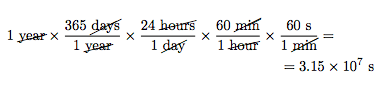Converting 1 year into seconds using the Factor-Label Method: Physically crossing out units that cancel out helps visualize the “leftover” unit(s).

So, when the units mile and hour are cancelled out and the arithmetic is done, 10 miles per hour converts to 4.47 meters per second.

A limitation of the factor-label method is that it can only convert between units that have a constant ratio that can be multiplied, or a multiplication factor. This method cannot be used between units that have a displacement, or difference factor. An example is the conversion between degrees Celsius and kelvins, or between Celsius and Fahrenheit. For these, it is best to use the specific conversion formulas.

For example, if you are planning a trip abroad in Spain and the weather forecast predicts the weather to be mostly cloudy and 16°C, you may want to convert the temperature into °F, a unit that you are more comfortable interpreting. In order to do this, you would need to know the conversion formula from Celsius to Fahrenheit. This formula is: [°F] = [°C] × 95 + 32.

[°F] = (16 × 95)+ 32

[°F] = 28.8 + 32

[°F] = 60.8

So you would then know that 16°C is equivalent to 60.8°F and be able to pack the right type of clothing to be comfortable.